Peano theorem

(diff) ← Older revision | Latest revision (diff) | Newer revision → (diff)

One of the existence theorems for solutions of an ordinary differential equation (cf. Differential equation, ordinary), established by G. Peano , and consisting in the following. Suppose one is given the differential equation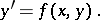(*)

If the functionis bounded and continuous in a region, then through each interior point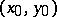of this region there passes at least one integral curve for (*). It may be that more than one integral curve passes through a certain point, e.g. for the equationthere exists an infinite set of integral curves passing through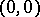: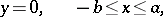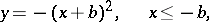whereandare arbitrary constants.

There are generalizations (including multi-dimensional ones) of Peano's theorem (see , ).

How to Cite This Entry:
Peano theorem. Encyclopedia of Mathematics. URL: http://encyclopediaofmath.org/index.php?title=Peano_theorem&oldid=14971
This article was adapted from an original article by M.I. Voitsekhovskii (originator), which appeared in Encyclopedia of Mathematics - ISBN 1402006098. See original article